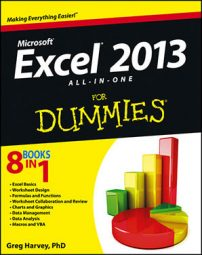##### Excel 2013 All-in-One For DummiesExcel 2013 offers far fewer Time functions when compared with the wide array of Date functions. Like the Date functions, however, the Time functions enable you to convert text entries representing times of day into time serial numbers so that you can use them in calculations.

The Time functions also include functions for combining different parts of a time into a single serial time number, as well as those for extracting the hours, minutes, and seconds from a single time serial number.

## NOW

The NOW function on the Date & Time command button’s drop-down menu gives you the current time and date based on your computer’s internal clock. You can use the NOW function to date- and time-stamp the worksheet. Like the TODAY function, NOW takes no arguments and is automatically recalculated every time you open the spreadsheet:

`=NOW()`

When you enter the NOW function in a cell, Excel puts the date before the current time. It also formats the date with the first Date format and the time with the 24-hour Time format. So, if the current date was September 15, 2012, and the current time was 10:53 a.m. at the moment when Excel calculates the NOW function, your cell would contain the following entry:

`9/15/2012 10:53`

Note that the combination Date/Time format that the NOW function uses is a custom number format. If you want to assign a different date/time to the date and time serial numbers returned by this function, you have to create your own custom number format and then assign it to the cell that contains the NOW function.

## TIME and TIMEVALUE

The TIME function on the Date & Time command button’s drop-down menu enables you to create a decimal number representing a time serial number, ranging from 0 (zero) to 0.99999999, representing time 0:00:00 (12:00:00 AM) to 23:59:59 (11:59:59 PM). You can use the TIME function to combine the hours, minutes, and seconds of a time into a single time serial number when these parts are stored in separate cells.

The TIME function takes the following arguments:

`TIME(hour,minute,second)`

When specifying the hour argument, you use a number between 0 and 23. (Any number greater than 23 is divided by 24, and the remainder is used as the hour value.) When specifying the minute and second arguments, you use a number between 0 and 59. (Any minute argument greater than 59 is converted into hours and minutes, just as any second argument greater than 59 is converted into hours, minutes, and seconds.)

For example, if cell A3 contains 4, cell B3 contains 37, and cell C3 contains 0, and you enter the following TIME function in cell D3:

`=TIME(A3,B3,C3)`

Excel enters 4:37 AM in cell D3. If you then assign the General number format to this cell (Ctrl+Shift+` or Ctrl+~), it would then contain the time serial number, 0.192361.

The TIMEVALUE function converts a time entered or imported into the spreadsheet as a text entry into its equivalent time serial number so that you can use it in time calculations. The TIMEVALUE function uses a single time_text argument as follows:

`TIMEVALUE(<i>time_text</i>)`

So, for example, if you put the following TIMEVALUE function in a cell to determine the time serial number for 10:35:25:

`=TIMEVALUE("10:35:25")`

Excel returns the time serial number 0.441262 to the cell. If you then assign the first Time number format to this cell, the decimal number appears as 10:35:25 a.m. in the cell.

## HOUR, MINUTE, and SECOND

The HOUR, MINUTE, and SECOND functions on the Date & Time command button’s drop-down menu enable you to extract specific parts of a time value in the spreadsheet. Each of these three Time functions takes a single serial_number argument that contains the hour, minute, or second that you want to extract.

So, for example, if cell B5 contains the time 1:30:10 p.m. (otherwise known as serial number 0.5626157) and you enter the following HOUR function in cell C5:

`=HOUR(B5)`

Excel returns 13 as the hour to cell C5 (hours are always returned in 24-hour time). If you then enter the following MINUTE function in cell D5:

`=MINUTE(B5)`

Excel returns 30 as the number of minutes to cell D5. Finally, if you enter the following SECOND function in cell E5:

`=SECOND(B5)`

Excel returns 10 as the number of seconds to cell E5.